# Linear Filters in Stream It Andrew A Lamb

• Slides: 41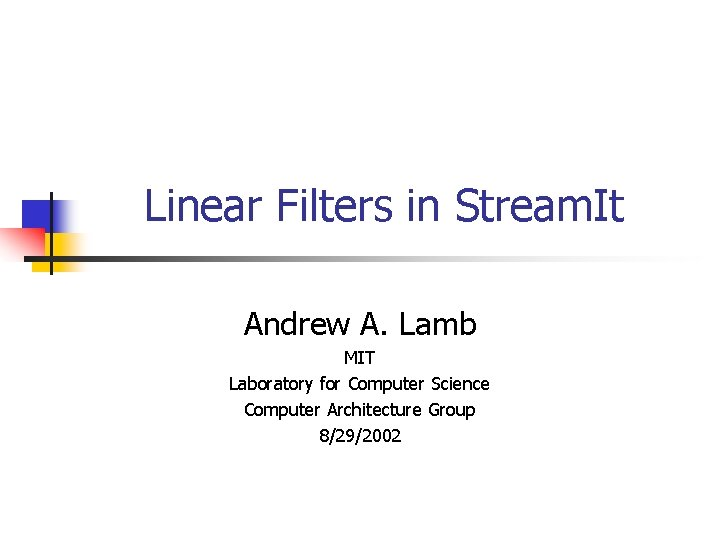Linear Filters in Stream. It Andrew A. Lamb MIT Laboratory for Computer Science Computer Architecture Group 8/29/2002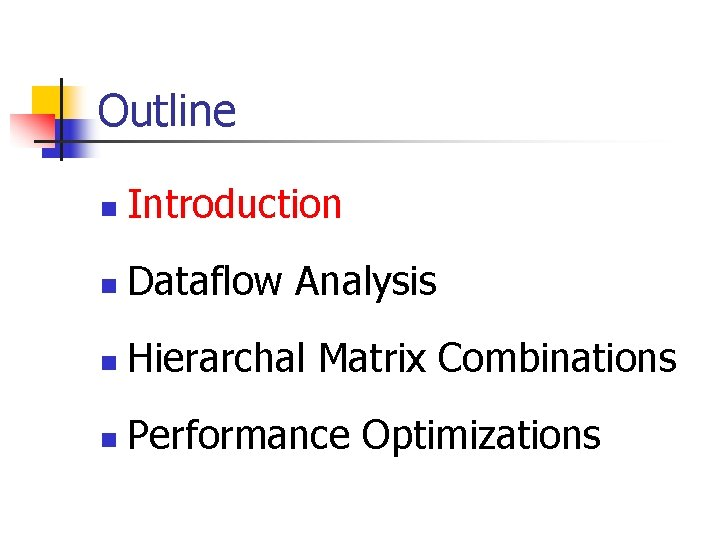Outline n Introduction n Dataflow Analysis n Hierarchal Matrix Combinations n Performance OptimizationsBasic Idea Filter A a=pop(); b=pop(); c=(a+b)/2 + 1; push(c); x Matrix Mult. y = x. A + b y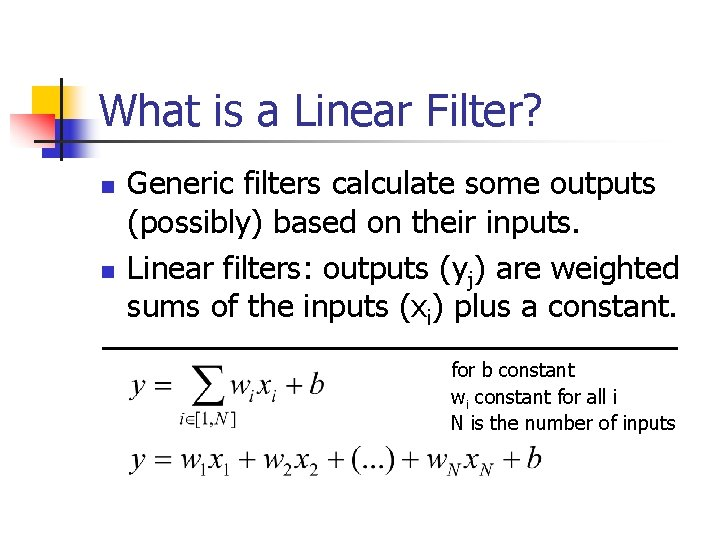What is a Linear Filter? n n Generic filters calculate some outputs (possibly) based on their inputs. Linear filters: outputs (yj) are weighted sums of the inputs (xi) plus a constant. for b constant wi constant for all i N is the number of inputsLinearity and Matricies n n Matrix multiply is exactly weighted sum We treat inputs (xi) and outputs (yj) as vectors of values (x, and y respectively) Filter is represented as a matrix of weights A and a vector of constants b Therefore, filter represents the equation y = x. A + b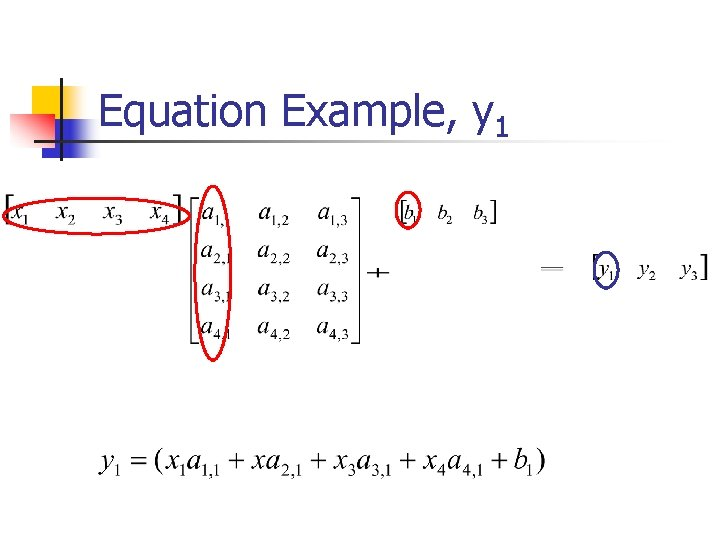Equation Example, y 1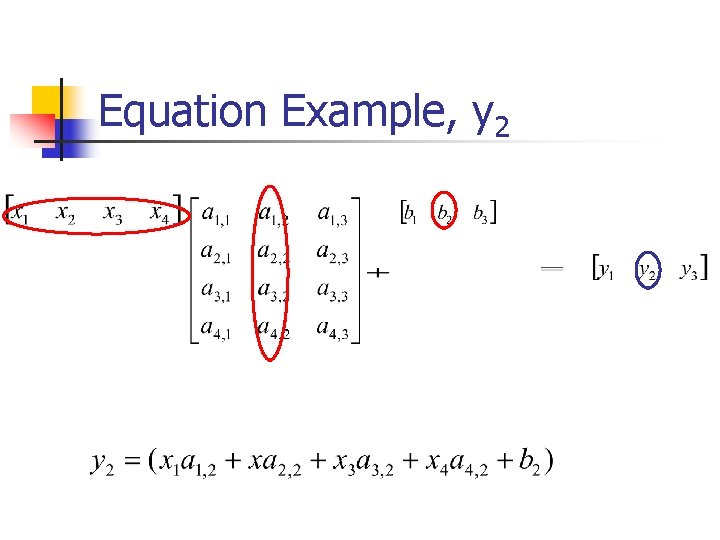Equation Example, y 2Equation Example, y 3Usefulness of Linearity n Not all filters compute linear functions n n push(pop()*pop()); Many fundamental DSP filters do n n DFT/FFT DCT Convolution/FIR Matrix Multiply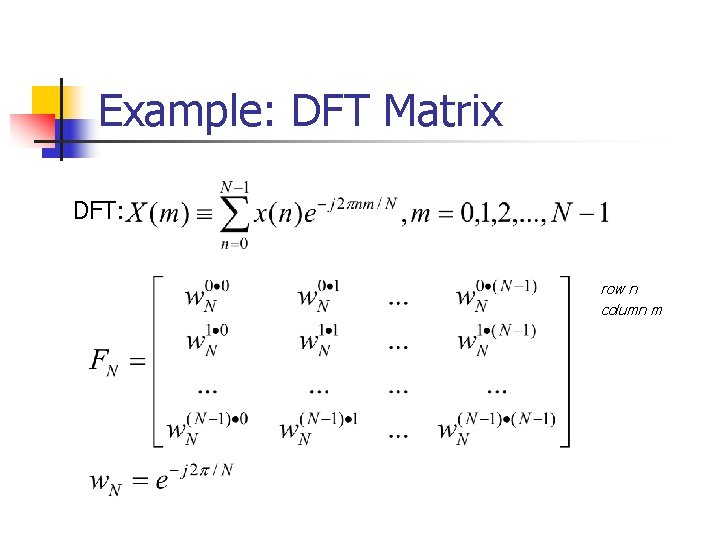Example: DFT Matrix DFT: row n column m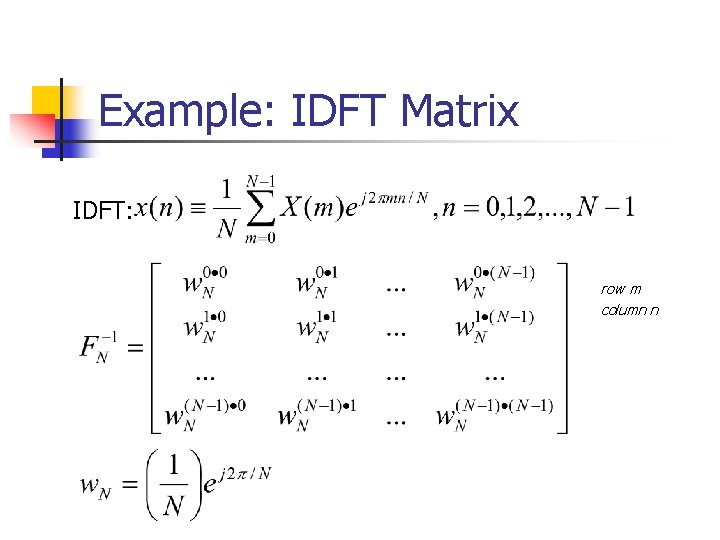Example: IDFT Matrix IDFT: row m column n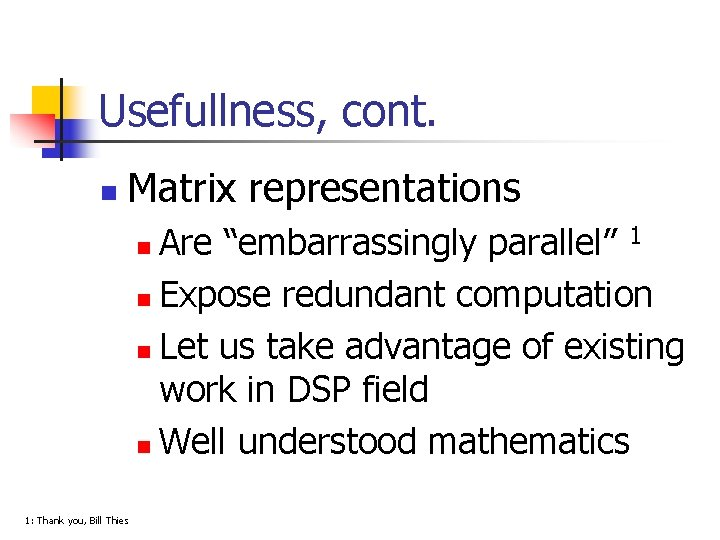Usefullness, cont. n Matrix representations Are “embarrassingly parallel” 1 n Expose redundant computation n Let us take advantage of existing work in DSP field n Well understood mathematics n 1: Thank you, Bill Thies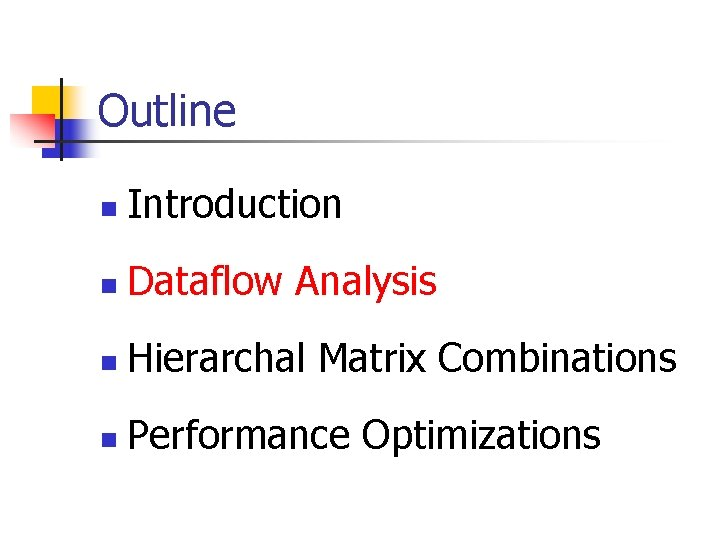Outline n Introduction n Dataflow Analysis n Hierarchal Matrix Combinations n Performance Optimizations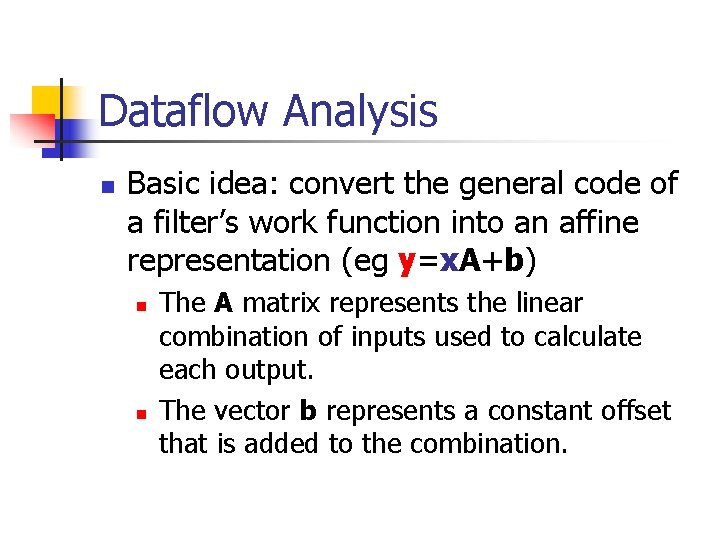Dataflow Analysis n Basic idea: convert the general code of a filter’s work function into an affine representation (eg y=x. A+b) n n The A matrix represents the linear combination of inputs used to calculate each output. The vector b represents a constant offset that is added to the combination.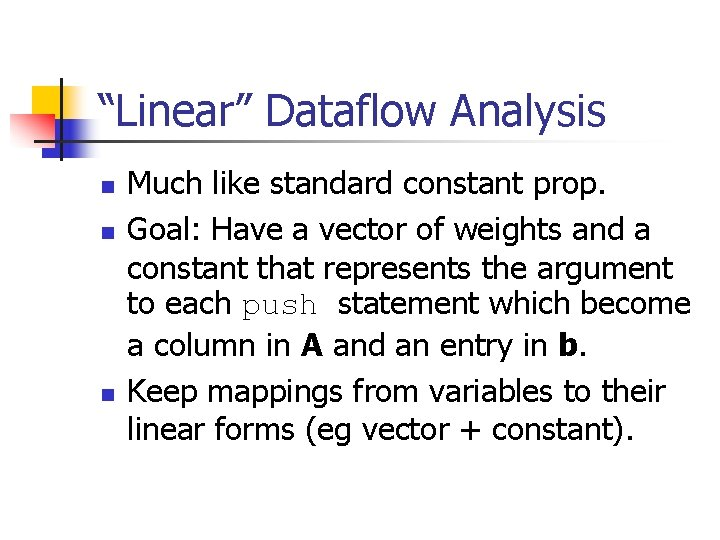“Linear” Dataflow Analysis n n n Much like standard constant prop. Goal: Have a vector of weights and a constant that represents the argument to each push statement which become a column in A and an entry in b. Keep mappings from variables to their linear forms (eg vector + constant).“Linear” Dataflow Analysis n Of course, we need the appropriate generating cases, eg n constants n pop/peek(x)“Linear” Dataflow Analysis n n Like const prop, confluence operator is set union. Need combination rules to handle things like multiplication and addition (vector add and constant scale)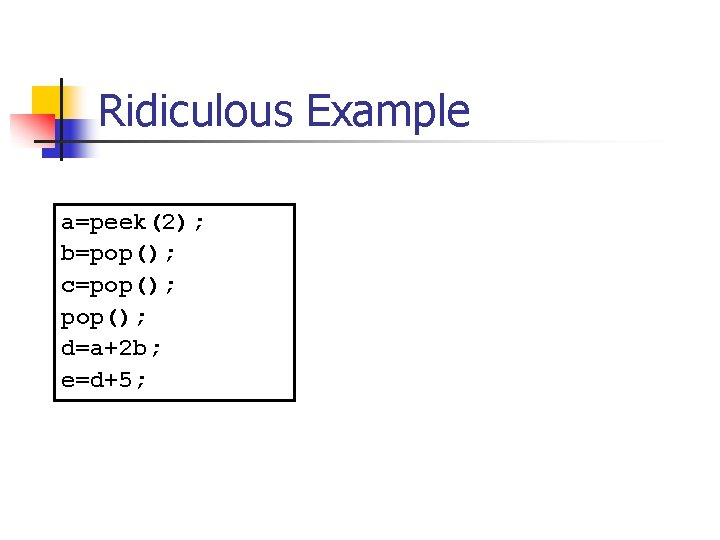Ridiculous Example a=peek(2); b=pop(); c=pop(); d=a+2 b; e=d+5;Ridiculous Example a=peek(2); b=pop(); c=pop(); d=a+2 b; e=d+5; a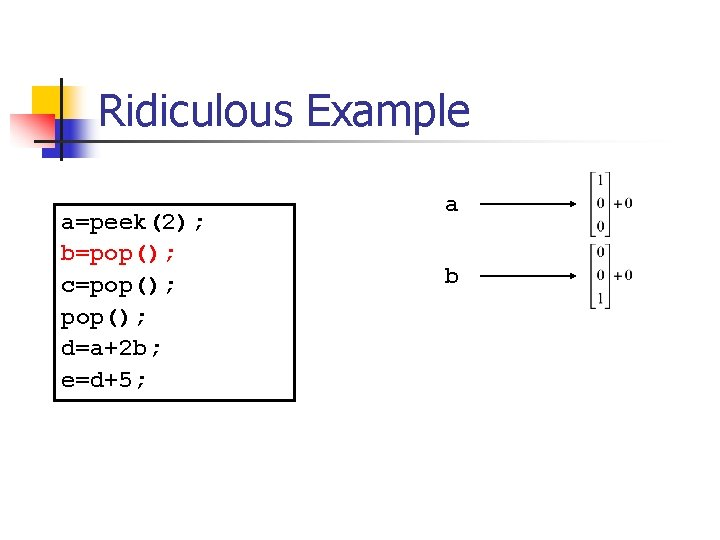Ridiculous Example a=peek(2); b=pop(); c=pop(); d=a+2 b; e=d+5; a b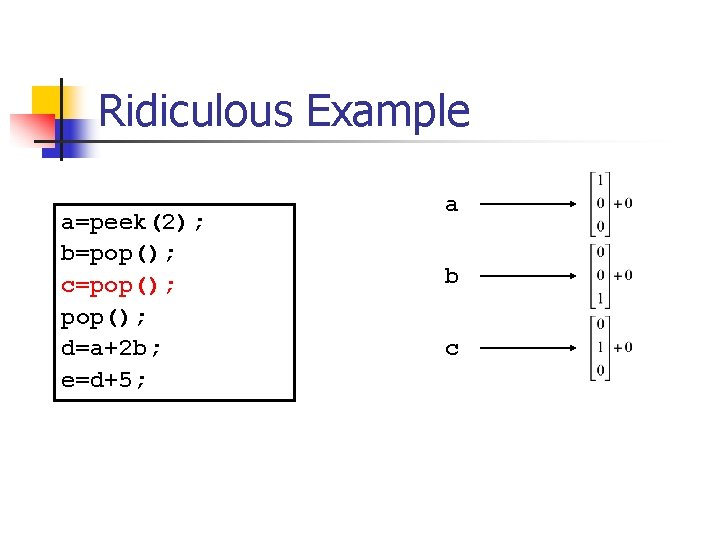Ridiculous Example a=peek(2); b=pop(); c=pop(); d=a+2 b; e=d+5; a b cRidiculous Example a=peek(2); b=pop(); c=pop(); d=a+2 b; e=d+5; a b c dRidiculous Example a=peek(2); b=pop(); c=pop(); d=a+2 b; e=d+5; a b c d e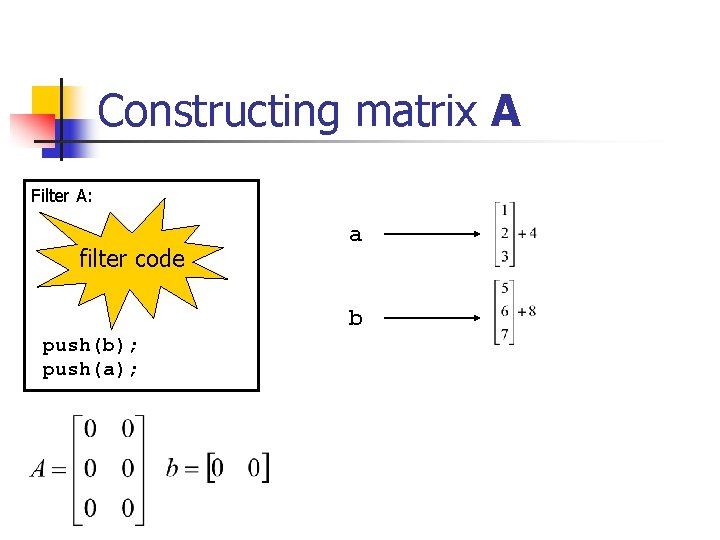Constructing matrix A Filter A: filter code a b push(b); push(a);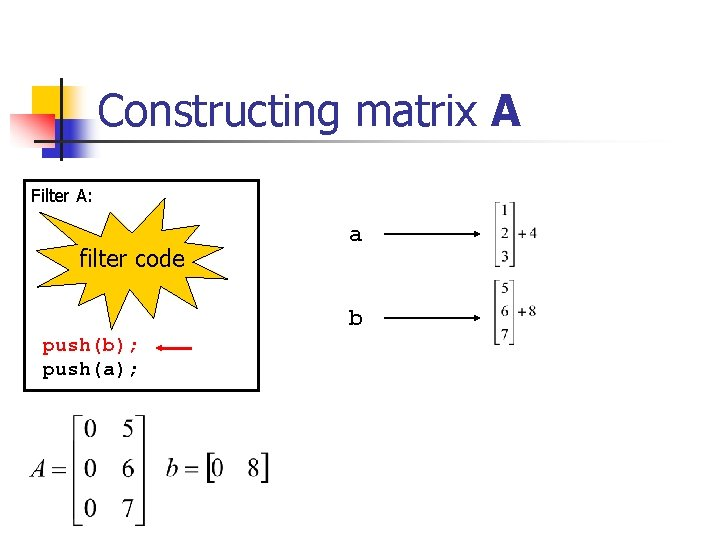Constructing matrix A Filter A: filter code a b push(b); push(a);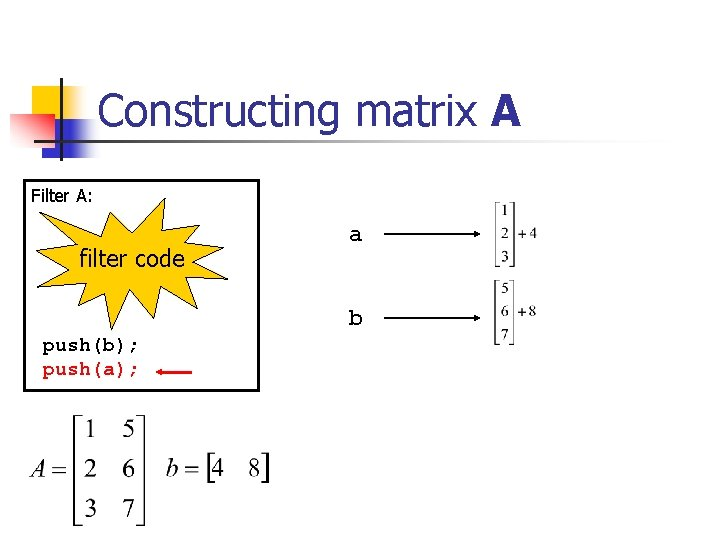Constructing matrix A Filter A: filter code a b push(b); push(a);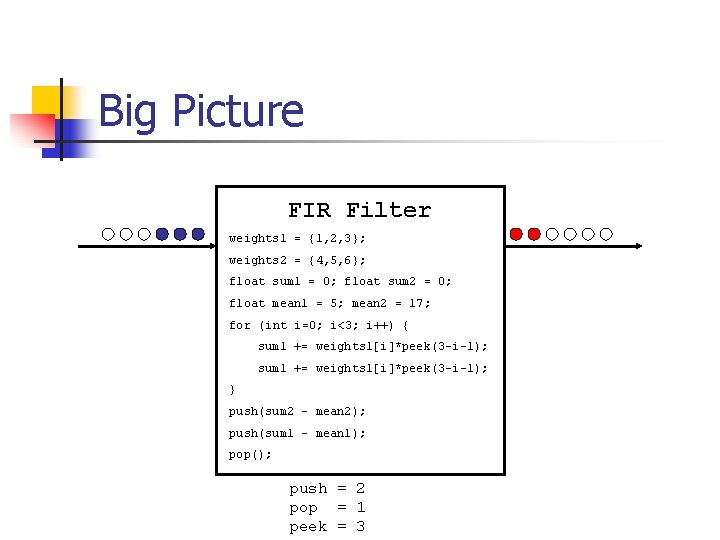Big Picture FIR Filter weights 1 = {1, 2, 3}; weights 2 = {4, 5, 6}; float sum 1 = 0; float sum 2 = 0; float mean 1 = 5; mean 2 = 17; for (int i=0; i<3; i++) { sum 1 += weights 1[i]*peek(3 -i-1); } push(sum 2 – mean 2); push(sum 1 – mean 1); pop(); push = 2 pop = 1 peek = 3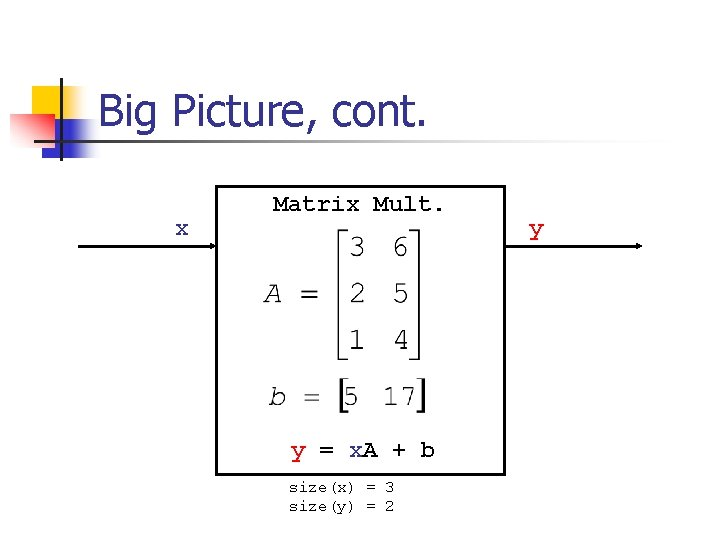Big Picture, cont. x Matrix Mult. y = x. A + b size(x) = 3 size(y) = 2 y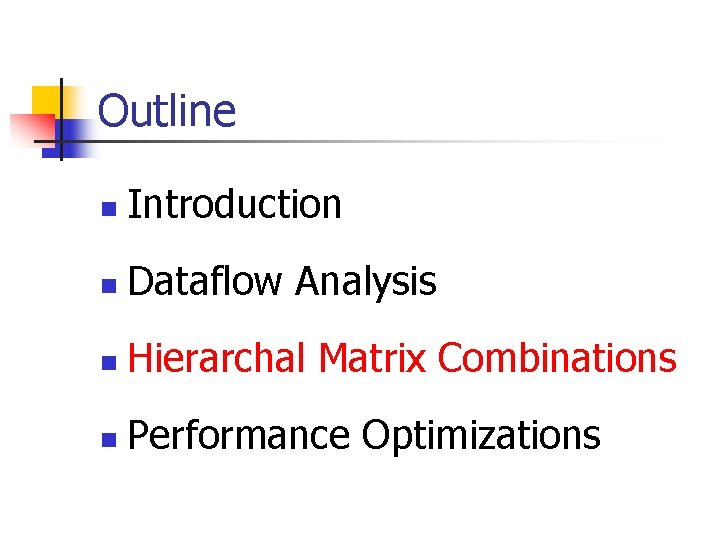Outline n Introduction n Dataflow Analysis n Hierarchal Matrix Combinations n Performance OptimizationsCombining Filters n n n Basic idea: combine pipelines, splitjoins (and possibly feedback loops) of linear filters together End up with a single large matrix representation The details are tricky in the general case (eg I am still working on them)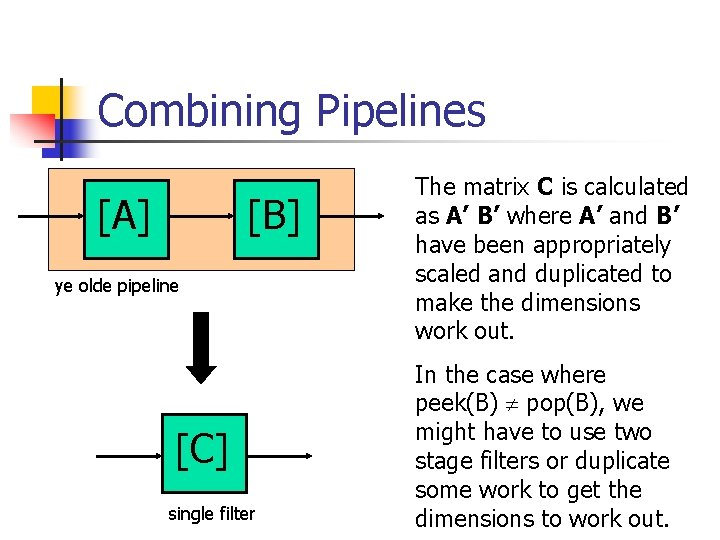Combining Pipelines [A] [B] ye olde pipeline [C] single filter The matrix C is calculated as A’ B’ where A’ and B’ have been appropriately scaled and duplicated to make the dimensions work out. In the case where peek(B) pop(B), we might have to use two stage filters or duplicate some work to get the dimensions to work out.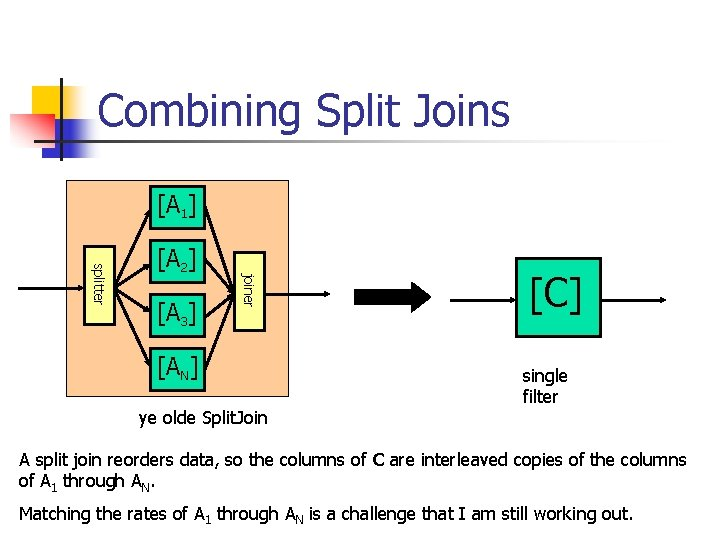Combining Split Joins [A 1] [A 3] joiner splitter [A 2] [AN] ye olde Split. Join [C] single filter A split join reorders data, so the columns of C are interleaved copies of the columns of A 1 through AN. Matching the rates of A 1 through AN is a challenge that I am still working out.Combining Feedback Loops splitter joiner [A] ? [B] ye olde Feedback. Loop It is unclear if we can do anything of use with a Feedback. Loop construct. Eigen values might give information about stability, but it is not clear if that is useful… more thought is needed.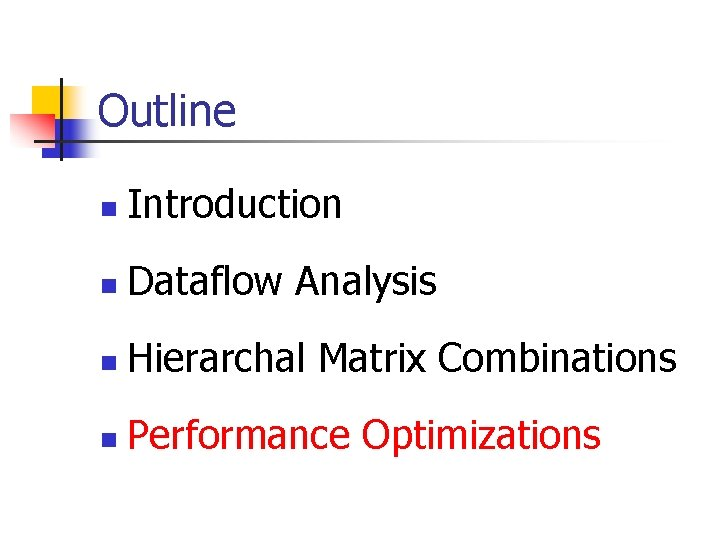Outline n Introduction n Dataflow Analysis n Hierarchal Matrix Combinations n Performance Optimizations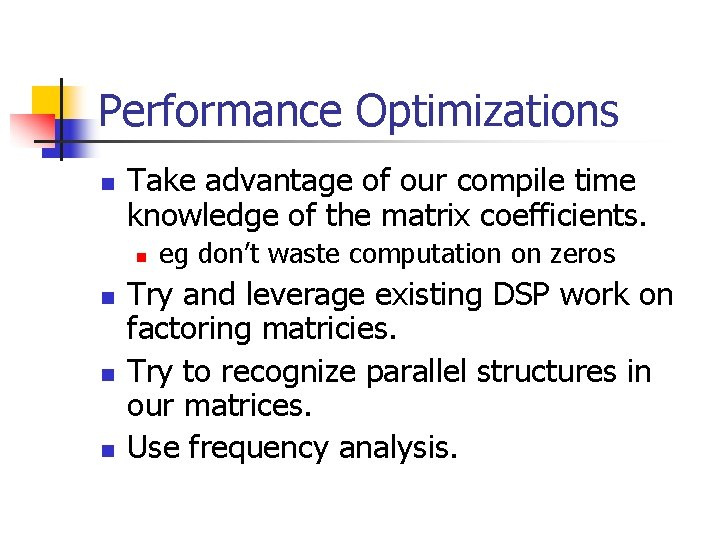Performance Optimizations n Take advantage of our compile time knowledge of the matrix coefficients. n n eg don’t waste computation on zeros Try and leverage existing DSP work on factoring matricies. Try to recognize parallel structures in our matrices. Use frequency analysis.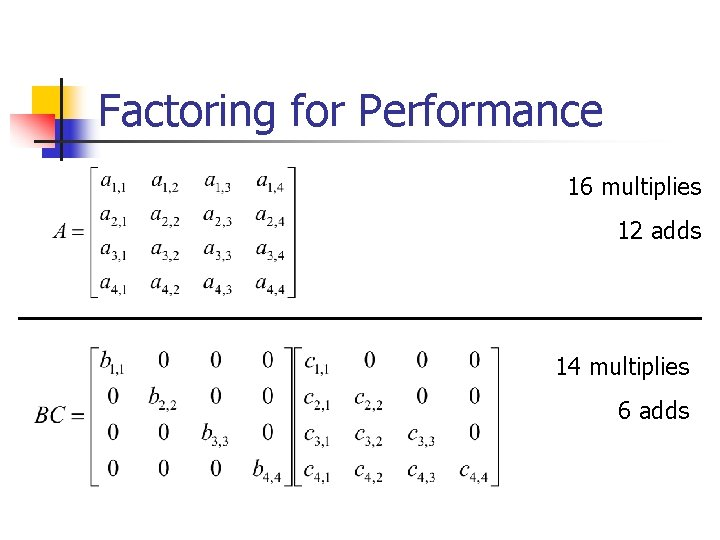SPL/SPIRAL n n Software package that will attempt to find a fast implementation signal processing algorithms described as matrices. It attempts to find a sparse factorization of an arbitrary matrix. It can automatically derive FFT (eg the Cooley-Turkey algorithm) from DFT definition. Claim that their performance is FFTW 1. See http: //www. fftw. orgRecognize Parallel Structure n n n We can go from Split. Join to matrix. Perhaps we can recognize the reverse transformation. Also, implement blocked matrix multiply to keep parallel resources busy.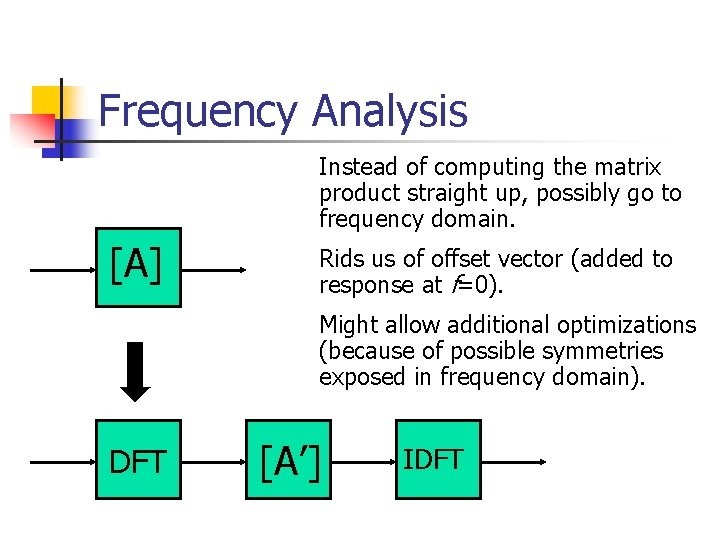Frequency Analysis Instead of computing the matrix product straight up, possibly go to frequency domain. [A] Rids us of offset vector (added to response at f=0). Might allow additional optimizations (because of possible symmetries exposed in frequency domain). DFT [A’] IDFTWork left to do Implementation of single filter analysis. n Combining hierarchical constructs. n Understand the math of automatic matrix factorizations (group theory). n Analyze frequency analysis. n Implement optimizations. n Get results. nQuestions for the Future n n Are there any other optimizations? Can we produce inverted matrices n n n 1. Thank you, BT programmer codes up transmitter and Stream. It automatically creates the receiver. 1 How many cycles of a “real” DSP application are spent computing linear functions? Can we combine the linear description of what happens inside a filter with the SARE representation of what is happening between them? (POPL paper)